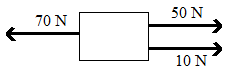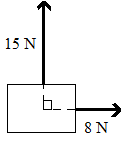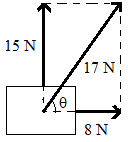# Resultant Force Formula

## Resultant Force Formulas - Solved Examples & Practice Questions

When two or more forces are acting on a body, then the total of all the forces which causes the resulting effect is the resultant force or net force. As force is a vector, we need to take the vector sum of all the forces to calculate the resultant. A suitable combination of several forces acting on a body may give a zero resultant causing no net effect.
Generally, if ${\vec F_1},\,\,{\vec F_2},\,\,{\vec F_3}\,\,....$are the forces acting on a body, their resultant force $\vec F$is given by:
$\vec F = {\vec F_1} + {\vec F_2} + \,\,{\vec F_3}\,\,....$
Depending on the number forces acting, the resultant can be obtained geometrically by applying triangle law, parallelogram law or polygon law of vector addition.
Note that the magnitudes of the forces cannot be added or subtracted unless they are collinear.

Example:
Find the resultant of the three forces shown in the figure.Solution:
Let F1, F2 and F3 be the forces with magnitudes 50 N, 10 N and 70 N. Also let the direction towards right is positive. Then;
F1 = 50 N, F2 = 10 N, and F3 = – 70 N
The resultant force: F = F1 + F2 + F3 = 50 + 10 – 70 = –10 N
F = – 10 N means, the resultant force is of magnitude 10 N, acting towards the left.

Example: Two friends are applying forces on a table as shown in the figure, in which direction will the table move?Solution:
The resultant force can be obtained by using parallelogram law of vectors.The net force has a magnitude of 17 N (using Pythagoras theorem. It is directed at an angle $\theta = {\tan ^{--1}}\left( {\frac{{15}}{8}} \right)$ with the force of 8 N. The table will move in this direction.

Question: Three forces of equal magnitudes are acting on a body. Then choose the correct statement.
Options:
(a) The resultant force can never be zero
(b) The resultant can be zero if all of them are collinear
(c) For zero resultant, one force should be opposite to the combination of the other two.
(d) The resultant of three equal forces is always zero.